# Classifying Triangles Calculator

Created by Julia Żuławińska
Reviewed by Arturo Barrantes
Last updated: Jun 09, 2022

The classifying triangles calculator can help you name a chosen triangle. Triangles have two types of names. With our calculator, you'll learn how to classify triangles by their sides. Then, you'll also find out how to name them based on the sizes of their angles.

## How to classify a triangle by its sides?

To classify a triangle by its sides, you need to check how many sides have equal length.

• ** Equilateral** triangle - has three equal sides (and three equal angles);
• Isosceles triangle - has two equal sides; and
• Scalene triangle - has no equal sides.

## How to classify a triangle by its angles?

There are three types of triangles based on their angles:

• If all of the triangle angles are less than 90°, then it's an acute triangle;
• If one of the angles measures more than 90°, it's an obtuse triangle; and
• If one of the angles is exactly 90°, then it's a right triangle.

## What are the two ways triangles are named?

Now you know that triangles can be classified by their sides or angles. A triangle can be equilateral, isosceles, or scalene, but also acute, obtuse, or right. When you try using our classifying triangles calculator, you will also find out that you can mix these names to give a more detailed description:

sides all equal all equal to 60°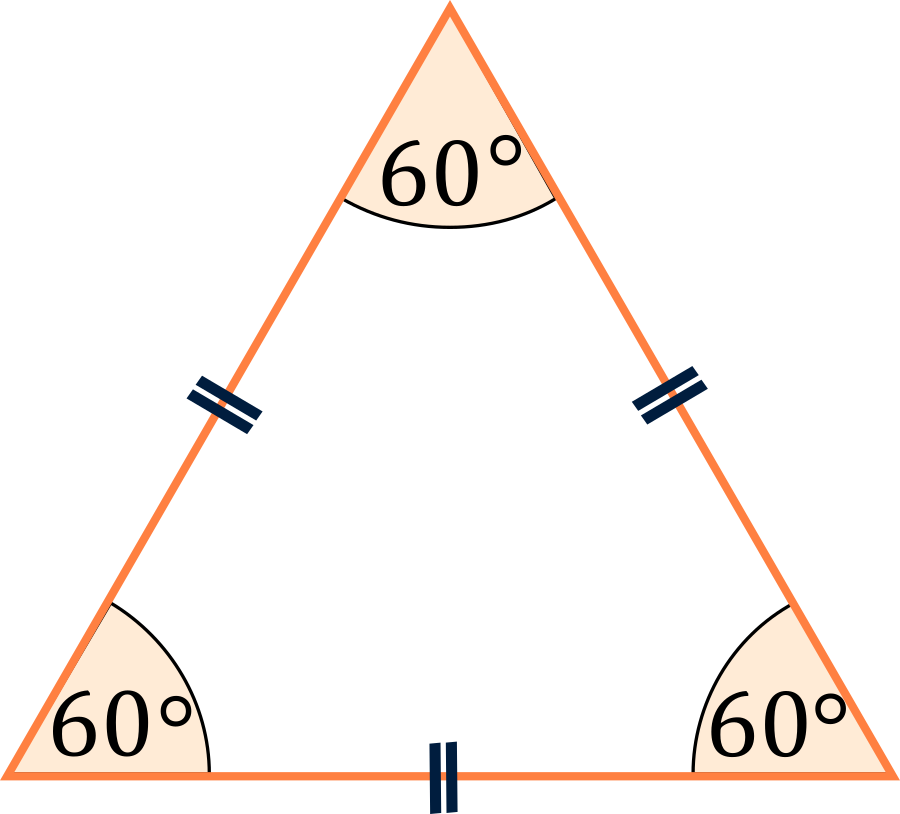• Acute scalene
sides all different all less than 90°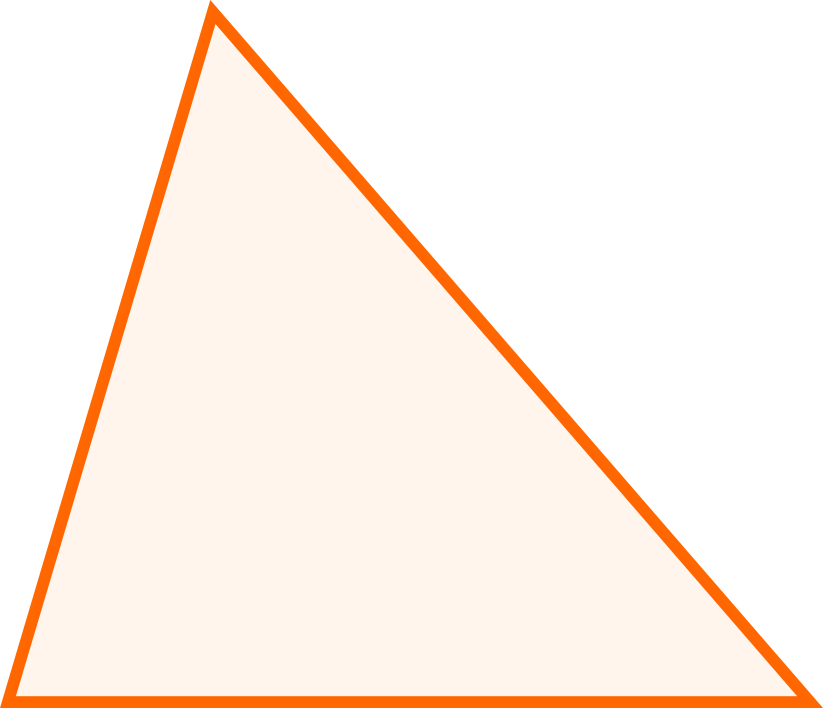• Obtuse scalene
sides all different one larger than 90°sides all different one equal to 90°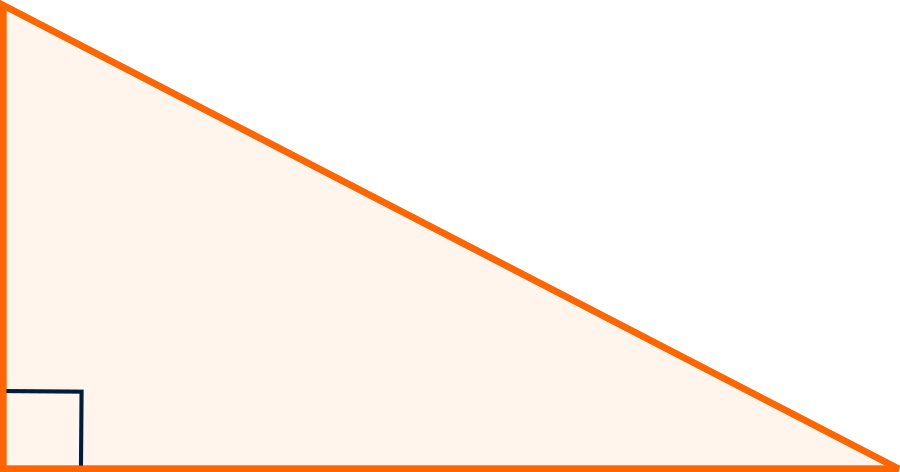sides two equal all less than 90° and two equal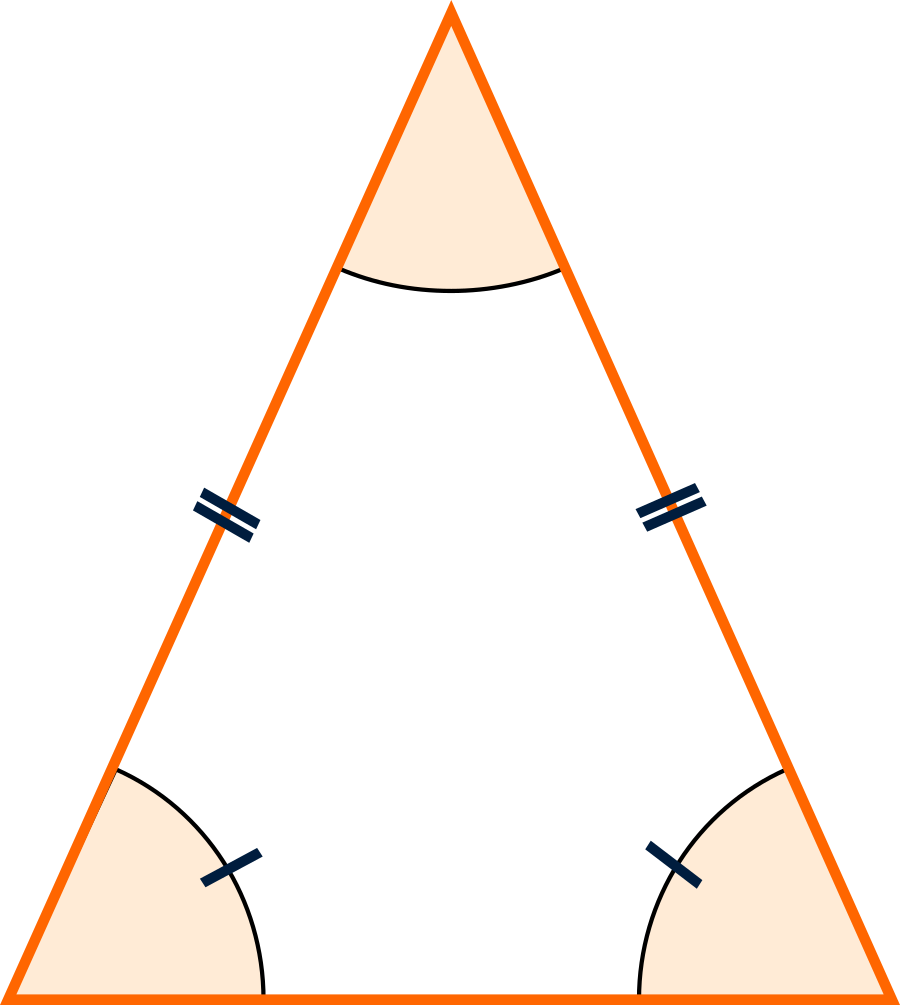• Obtuse isosceles
sides two equal one larger than 90° and the other two equal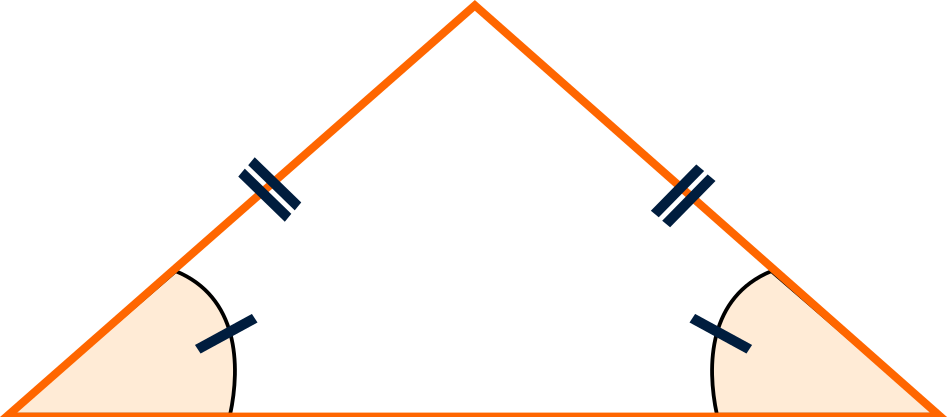• Right isosceles
sides two equal one equal to 90° and the other two equal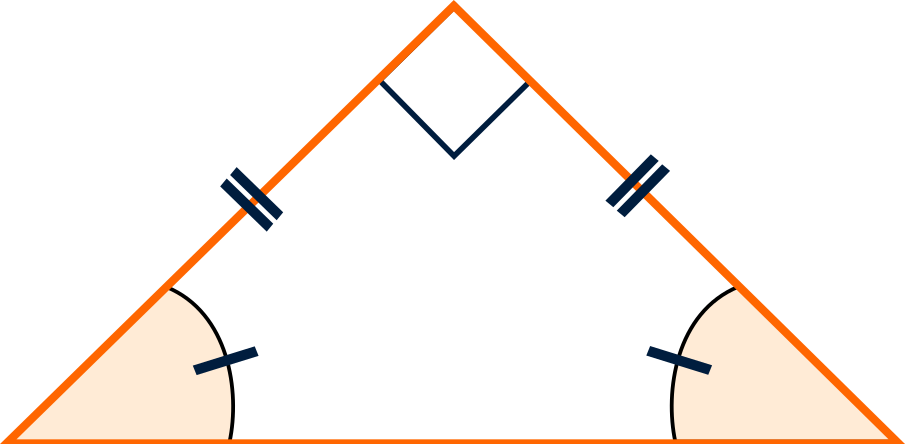💡 Did you know that if you know the length of the sides of a triangle, you can also determine the size of its angles? However, it doesn't work the other way around. You can't find the sides of a triangle from its angles.

## How to use the classifying triangles calculator?

With our calculator, you can find out the type of your triangle based on your parameters. You can input:

• Three sides;
• Angle and two sides; or
• Two angles.

Based on that, the classifying triangles calculator will:

• Give you other parameters that it could determine about your triangle;
• Tell you the type of your triangle, and
• Show you an example of what your type of triangle might look like.

## FAQ

### How to find the type of triangle with side lengths?

To find the type of a triangle, you need to check how many of its sides have the same length.

• If all of the sides are different, it's a scalene triangle;
• If all of the sides are the same, it's an equilateral triangle; and
• If two sides are the same, and one is different, is an isosceles triangle.

### What triangle has all equal sides?

An equilateral triangle has all three equal sides. It also always has all equal angles, and all of them measure 60 degrees.

### What makes a triangle acute?

A triangle is acute when all of its angles is less than 90 degrees. For example, a triangle with angles of 80, 70, and 30 degrees is an acute triangle (remember that the sum of angles of a triangle is always 180 degrees).

Julia Żuławińska
Given
angle and 2 sides
Angle selection
αα
deg
a
in
b
in
c
in
β
deg
γ
deg
People also viewed…

### Inverse matrix

The inverse matrix calculator will check if the square matrix you give it has an inverse, and, if it does, will calculate it in a few easy steps.

### Right triangle

Right triangle calculator helps you find the sides and area of a right triangle.

### Social Media Time Alternatives

Check what you could have accomplished if you get out of your social media bubble.